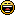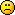<![CDATA[Math Is Fun Forum / help me! Q=D={x|x is an integer such that x^2=2)]]> 2014-05-21T02:06:07Z FluxBB http://www.mathisfunforum.com/viewtopic.php?id=20921 <![CDATA[Re: help me! Q=D={x|x is an integer such that x^2=2)]]> You need another colon after the last l

]]>
http://www.mathisfunforum.com/profile.php?id=95904 2014-05-21T02:06:07Z http://www.mathisfunforum.com/viewtopic.php?pid=315402#p315402
<![CDATA[Re: help me! Q=D={x|x is an integer such that x^2=2)]]>]]>
http://www.mathisfunforum.com/profile.php?id=97578 2014-05-21T01:03:31Z http://www.mathisfunforum.com/viewtopic.php?pid=315386#p315386
<![CDATA[Re: help me! Q=D={x|x is an integer such that x^2=2)]]> Theorem: All Questions are worthy

Proof: First, we have already seen that Bob has produced a lot of worthy questions, therefore worthy questions exist.

Now, Let us on the contrary assume that there exists some questions which are non-worthy.

We now have seen that there are questions of variable worth.

List all questions that exist in order of their worth. Clearly, this list is countable. There must be a question that is the worthiest of all non-worthy question.

But this is again a worthy subject to study. hence, the question must be interesting too.

So, that question is not non-worthy. This process can be repeated countable times to prove that all of them in the list are worthy.

QED

I don't understand.  I thought if x was furthest when measured in inches, it was also furthest when measured in kilometres, etc.

He was asking about what DistanceFunction to use. You can have Euclidean Distance, Chess Board Distance, Hamming Distance, etc.

]]>
http://www.mathisfunforum.com/profile.php?id=95904 2014-05-21T00:31:01Z http://www.mathisfunforum.com/viewtopic.php?pid=315378#p315378
<![CDATA[Re: help me! Q=D={x|x is an integer such that x^2=2)]]> Of course, the total amount of usually considered axioms are in the hundreds.

]]>
http://www.mathisfunforum.com/profile.php?id=97578 2014-05-20T23:26:43Z http://www.mathisfunforum.com/viewtopic.php?pid=315370#p315370
<![CDATA[Re: help me! Q=D={x|x is an integer such that x^2=2)]]> Stefy wrote:

...which measure for distance...

I don't understand.  I thought if x was furthest when measured in inches, it was also furthest when measured in kilometres, etc.

So that needs further explanation for me.

Here's what wiki says:

http://en.wikipedia.org/wiki/Atlas_Mountains

http://en.wikipedia.org/wiki/Mount_Everest

http://en.wikipedia.org/wiki/Chimborazo

As I asked 'furthest from the centre' I think it's Chimborazo.

As for 9 axioms of fields; yes 9; but you cannot do any number theory without the axioms of set theory and logic too.

eg

def. 1 + 1 = 2
def  1 + 2 = 3
def  1 + 3 = 4

Theorem:

2 + 2 = 4

2 + 2 = (1 + 1) + 2                        (using substitution ... not one of 9)
= 1 + ( 1 + 2)                       ( associativity)
= 1 + 3                                 (using substitution)
= 4                                       (using substitution)

So you cannot do even simple arithmetic with additional axioms.

Bob

]]>
http://www.mathisfunforum.com/profile.php?id=67694 2014-05-20T22:07:04Z http://www.mathisfunforum.com/viewtopic.php?pid=315369#p315369
<![CDATA[Re: help me! Q=D={x|x is an integer such that x^2=2)]]> I was talking about the field axioms.

(3) There exists an additive identity 0.
(4) Existence of additive inverses (opposites).
(5) Multiplication is commutative.
(6) Multiplication is associative.
(7) There exists a multiplicative identity 1.
(8) Existence of multiplicative inverses (except for 0).
(9) Distributivity of multiplication over addition.

Speaking of cosmology, I am planning to have a career in quantum cosmology.

]]>
http://www.mathisfunforum.com/profile.php?id=97578 2014-05-20T20:37:11Z http://www.mathisfunforum.com/viewtopic.php?pid=315362#p315362
<![CDATA[Re: help me! Q=D={x|x is an integer such that x^2=2)]]> I do not agree with either answer to Q3. You have not defined which measure for distance we are using, so I will say that it's probably Atlas mountain.

I'm not sure hiw many axiims there are in math, though. I know that we have 9 in geometry.

]]>
http://www.mathisfunforum.com/profile.php?id=118786 2014-05-20T20:32:38Z http://www.mathisfunforum.com/viewtopic.php?pid=315361#p315361
<![CDATA[Re: help me! Q=D={x|x is an integer such that x^2=2)]]> ShivamS wrote:

There are only 9 axioms. Axioms outside of mathematics do not exist.

Hhmmm.  This is another theory ???

(1) 9 axioms ?  Please list them.

(2) Kinetic theory of gases for a start.  Oh yes, I believe cosmologists may have a few too.  What about Descartes ?  "I think therefore I am."

Bob

Well done for the answer to Q3.]]>
http://www.mathisfunforum.com/profile.php?id=67694 2014-05-20T20:20:16Z http://www.mathisfunforum.com/viewtopic.php?pid=315357#p315357
<![CDATA[Re: help me! Q=D={x|x is an integer such that x^2=2)]]> bob bundy wrote:

Often the case, but not essential.  But just to satisfy you; here are some worthy questions:

(1) What is bobbym's real age ?

(2) What is your favourite colour ?

(3) Where on Earth is the place that is furthest from the centre of the Earth ?

(4) Is this question worthy ?

Can you give an example of a question that is not worthy ?  And even if you can, you would be wrong (sorry) because your example breaks the first axiom of the theory.

Bob

1. 12

2. I do not know.

3. Of course not. I know enough physics (this pops up in geophysics) to know that it is Chimborazo in Ecuador.

Axioms outside of mathematics do not exist.]]>
http://www.mathisfunforum.com/profile.php?id=97578 2014-05-20T19:28:02Z http://www.mathisfunforum.com/viewtopic.php?pid=315352#p315352
<![CDATA[Re: help me! Q=D={x|x is an integer such that x^2=2)]]> Btw.  The answer to Q3 is not Mount Everest.

B

]]>
http://www.mathisfunforum.com/profile.php?id=67694 2014-05-20T18:50:25Z http://www.mathisfunforum.com/viewtopic.php?pid=315351#p315351
<![CDATA[Re: help me! Q=D={x|x is an integer such that x^2=2)]]> ShivamS wrote:

A theory is usually supported by some empirical evidence.

Often the case, but not essential.  But just to satisfy you; here are some worthy questions:

(1) What is bobbym's real age ?

(2) What is your favourite colour ?

(3) Where on Earth is the place that is furthest from the centre of the Earth ?

(4) Is this question worthy ?

Can you give an example of a question that is not worthy ?  And even if you can, you would be wrong (sorry) because your example breaks the first axiom of the theory.

Bob

]]>
http://www.mathisfunforum.com/profile.php?id=67694 2014-05-20T18:49:16Z http://www.mathisfunforum.com/viewtopic.php?pid=315350#p315350
<![CDATA[Re: help me! Q=D={x|x is an integer such that x^2=2)]]> Wait... Who said I cannot prove it?

]]>
http://www.mathisfunforum.com/profile.php?id=95904 2014-05-20T16:20:06Z http://www.mathisfunforum.com/viewtopic.php?pid=315316#p315316
<![CDATA[Re: help me! Q=D={x|x is an integer such that x^2=2)]]> Not really. Number theory, for example.

]]>
http://www.mathisfunforum.com/profile.php?id=118786 2014-05-20T14:00:45Z http://www.mathisfunforum.com/viewtopic.php?pid=315248#p315248
<![CDATA[Re: help me! Q=D={x|x is an integer such that x^2=2)]]> A theory is usually supported by some empirical evidence.

]]>
http://www.mathisfunforum.com/profile.php?id=97578 2014-05-20T13:06:08Z http://www.mathisfunforum.com/viewtopic.php?pid=315182#p315182
<![CDATA[Re: help me! Q=D={x|x is an integer such that x^2=2)]]> We have an axiom:

Agnishom wrote:

Axiom: All questions are worthy.

Bob

]]>
http://www.mathisfunforum.com/profile.php?id=67694 2014-05-20T13:05:19Z http://www.mathisfunforum.com/viewtopic.php?pid=315181#p315181